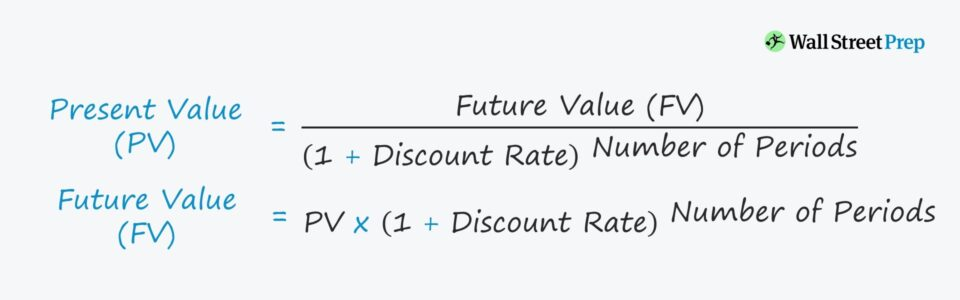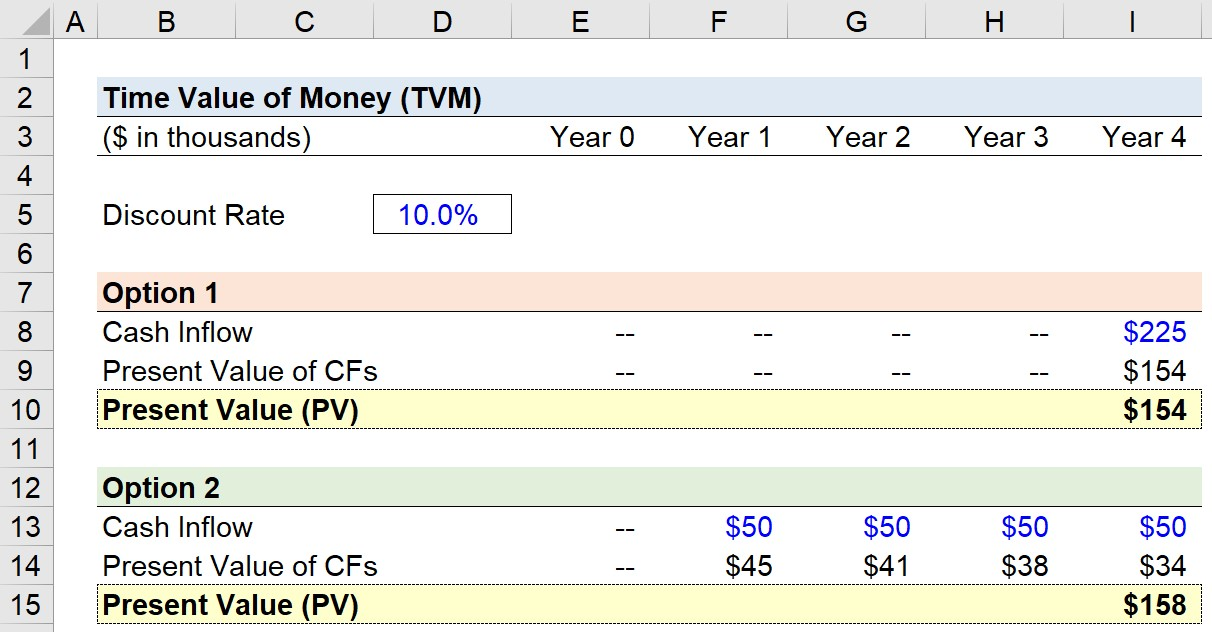Welcome to Wall Street Prep! Use code at checkout for 15% off.# Time Value of Money (TVM)

Guide to Understanding the Time Value of Money (TVM)## How to Calculate Time Value of Money (TVM)?

Under the time value of money (TVM) concept, a dollar received today is worth more than a dollar received at a later date — which is one of the most fundamental concepts in corporate finance.

In short, receiving money today is preferable (i.e. more valuable) than receiving the same amount of money on a later date.

There are two main reasons that underpin the TVM theory:

1. Opportunity Cost: If you have capital on hand currently, the funds could be used to invest into other projects to achieve a higher return — i.e. the “opportunity cost” of the money.
2. Inflation: There are risks to consider such as inflation or the probability that the company in question might go bankrupt in the future — i.e. future uncertainty should be costlier than the lower risks identified on the present date.

Since money tends to decline in value over time due to factors such as inflation, the purchasing power of money also decreases.

With that said, cash flows received in the future (and with increased uncertainty) are worth less than the present value (PV) of the cash flows.

If you risk one dollar in an investment, you should reasonably expect gains of more than solely your initial one-dollar contribution as a return.

For each incremental unit of risk you take on, you should expect a proportionally higher return in exchange.

## Why is the Time Value of Money Important?

The time value of money (TVM) matter because it serves as the basis of the net present value (NPV) calculation.

Briefly, suppose there are two investment options, as outlined below:

• Option 1 → In the first option, you can receive \$10,000 right now on the current date.
• Option 2 → In the second option, you can receive \$11,000, however, it’ll take one year before the funds are received.

To pick the “right” option rationally, you must consider the time value of money, which is essentially the required rate of return (i.e. cost of capital).

In this example, \$11,000 is 10% greater than \$10,000 — this serves as the minimum required rate of return if you would be indifferent between these investment options.

For the second option to make sense from a monetary perspective, the returns should exceed that of the 1st option, i.e. if you receive the \$10,000 on the present date and receive a return >10%, you should pick the first option, as it is more profitable.

## Time Value of Money Formula (TVM)

The formula for the time value of money, from the perspective of the current date, is as follows:\

Present Value (PV) = FV ÷ [1 +( i ÷ n) ^(n × t)

Where:

• PV = Present Value
• FV = Future Value
• i = Annual Rate of Return (Interest Rate)
• n = Number of Compounding Periods Each Year
• t = Number of Years

Alternatively, to calculate the future value given the present value, the formula used is:

Future Value (FV) = PV × [1 + (i ÷ n)] ^ (n × t)

In both formulas, “i” represents the rate of interest on comparable investments.

## Present Value and Future Value Calculation Example

For instance, if the present value (PV) of an investment is \$10 million, and the amount is invested at a rate of return of 10% for one year, the future value (FV) is equal to:

• FV = \$10 million * [1 + (10% / 1] ^ (1 × 1) = \$11 million

Moreover, using the same formula as above, we can calculate the future value (FV) assuming quarterly compound interest — i.e. 4.0x times a year:

Thus, the calculation for our example is as follows:

• FV = \$10 million * [1 + (10% / 4)] ^ (4 × 1) = \$11.04 million

## Time Value of Money Calculator (TVM)

We’ll now move to a modeling exercise, which you can access by filling out the form below.Submitting...

## TVM Calculation Example

Suppose you’re offered the following two options to pick from:

• Option 1: Receive \$225,000 in Year 4
• Option 2: Receive \$50,000 from Year 1 to Year 4

The determinant of which option is more profitable is the time value of money (TVM).

If we assume a 10% discount rate, which option should you proceed with?

For both option 1 and option 2, we’ll list out the cash inflow for each year.

While option 1 consists of a one-time payment of \$225,000, option 2 consists of four payments of \$50,000.

The formula for discounting each cash flow is the future value (FV) divided by (1 + discount rate), which is then raised to the power of the period number.

Once completed for each year, the sum of the discounted cash flows equals the present value of the option, i.e. how much the future cash flows are worth on the present date.

• Option 1 = \$154,000
• Option 2 = \$158,000

In our simple example, option 2 is worth more than option 1. But of course, there are far more considerations in reality that can complicate the decision-making process.Step-by-Step Online Course

### Everything You Need To Master Financial Modeling

Enroll in The Premium Package: Learn Financial Statement Modeling, DCF, M&A, LBO and Comps. The same training program used at top investment banks.Inline FeedbacksLearn Financial Modeling Online

Everything you need to master financial and valuation modeling: 3-Statement Modeling, DCF, Comps, M&A and LBO.

X

The Wall Street Prep Quicklesson Series

7 Free Financial Modeling Lessons

Get instant access to video lessons taught by experienced investment bankers. Learn financial statement modeling, DCF, M&A, LBO, Comps and Excel shortcuts.